# How to install

visdat is available on CRAN

``install.packages("visdat")``

If you would like to use the development version, install from github with:

``````# install.packages("devtools")
devtools::install_github("ropensci/visdat")``````

# What does visdat do?

Initially inspired by `csv-fingerprint`, `vis_dat` helps you visualise a dataframe and “get a look at the data” by displaying the variable classes in a dataframe as a plot with `vis_dat`, and getting a brief look into missing data patterns using `vis_miss`.

`visdat` has 6 functions:

• `vis_dat()` visualises a dataframe showing you what the classes of the columns are, and also displaying the missing data.

• `vis_miss()` visualises just the missing data, and allows for missingness to be clustered and columns rearranged. `vis_miss()` is similar to `missing.pattern.plot` from the `mi` package. Unfortunately `missing.pattern.plot` is no longer in the `mi` package (as of 14/02/2016).

• `vis_compare()` visualise differences between two dataframes of the same dimensions

• `vis_expect()` visualise where certain conditions hold true in your data

• `vis_cor()` visualise the correlation of variables in a nice heatmap

• `vis_guess()` visualise the individual class of each value in your data

• `vis_value()` visualise the value class of each cell in your data

• `vis_binary()` visualise the occurrence of binary values in your data

## Code of Conduct

Please note that the visdat project is released with a Contributor Code of Conduct. By contributing to this project, you agree to abide by its terms.

# Examples

## Using `vis_dat()`

Let’s see what’s inside the `airquality` dataset from base R, which contains information about daily air quality measurements in New York from May to September 1973. More information about the dataset can be found with `?airquality`.

``````library(visdat)

vis_dat(airquality)``````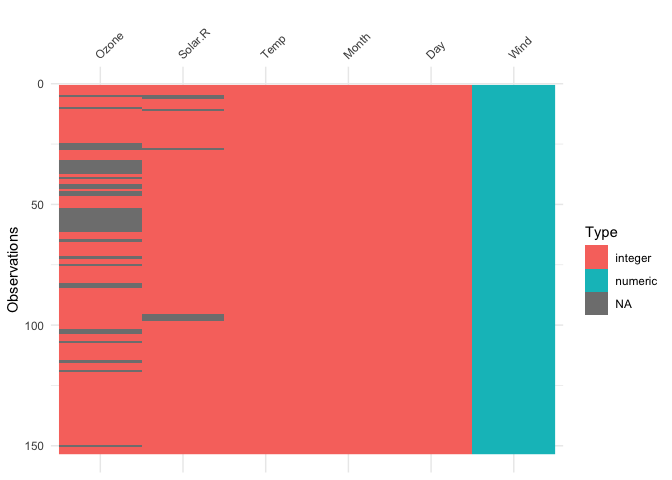The plot above tells us that R reads this dataset as having numeric and integer values, with some missing data in `Ozone` and `Solar.R`. The classes are represented on the legend, and missing data represented by grey. The column/variable names are listed on the x axis.

## Using `vis_miss()`

We can explore the missing data further using `vis_miss()`:

``vis_miss(airquality)``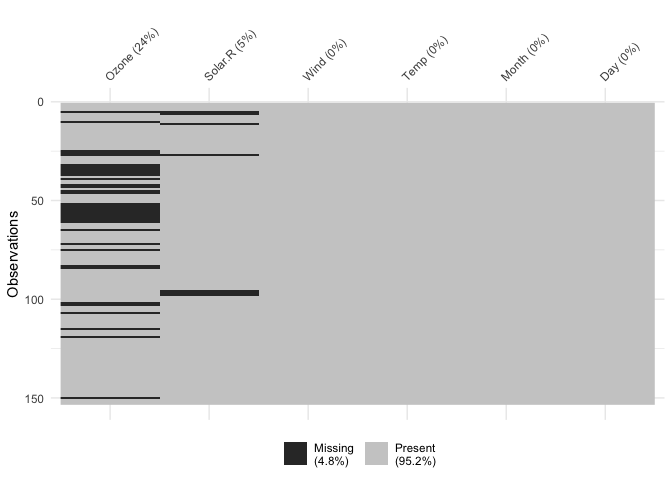Percentages of missing/complete in `vis_miss` are accurate to 1 decimal place.

You can cluster the missingness by setting `cluster = TRUE`:

``````vis_miss(airquality,
cluster = TRUE)``````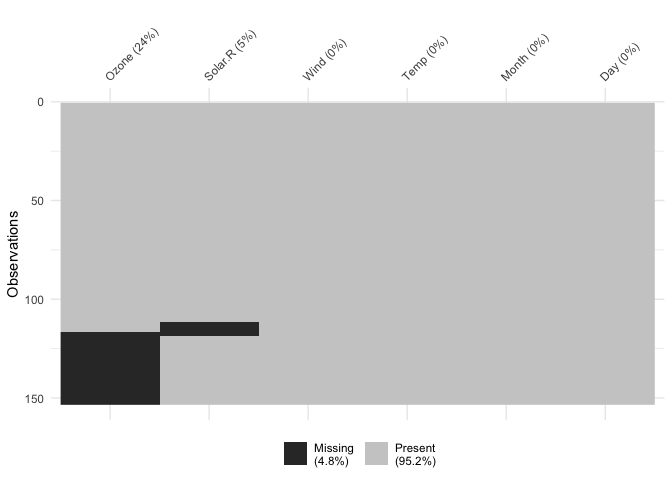Columns can also be arranged by columns with most missingness, by setting `sort_miss = TRUE`:

``````vis_miss(airquality,
sort_miss = TRUE)```````vis_miss` indicates when there is a very small amount of missing data at <0.1% missingness:

``````test_miss_df <- data.frame(x1 = 1:10000,
x2 = rep("A", 10000),
x3 = c(rep(1L, 9999), NA))

vis_miss(test_miss_df)``````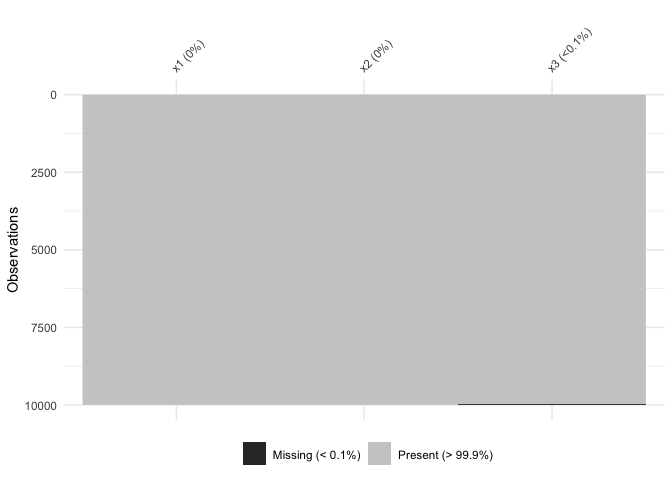`vis_miss` will also indicate when there is no missing data at all:

``vis_miss(mtcars)``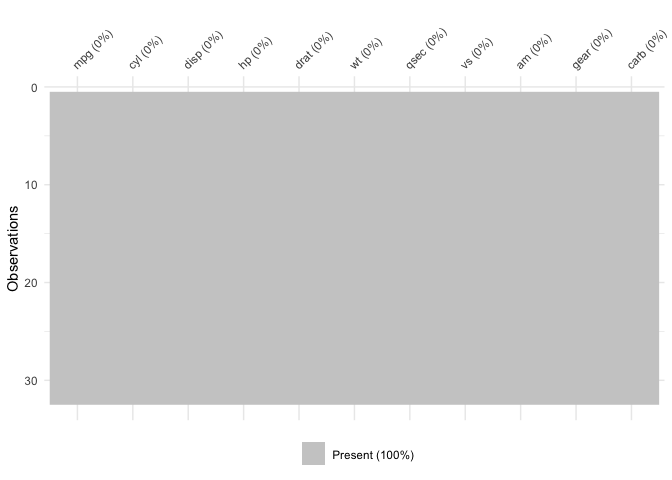To further explore the missingness structure in a dataset, I recommend the `naniar` package, which provides more general tools for graphical and numerical exploration of missing values.

## Using `vis_compare()`

Sometimes you want to see what has changed in your data. `vis_compare()` displays the differences in two dataframes of the same size. Let’s look at an example.

Let’s make some changes to the `chickwts`, and compare this new dataset:

``````set.seed(2019-04-03-1105)
chickwts_diff <- chickwts
chickwts_diff[sample(1:nrow(chickwts), 30),sample(1:ncol(chickwts), 2)] <- NA

vis_compare(chickwts_diff, chickwts)``````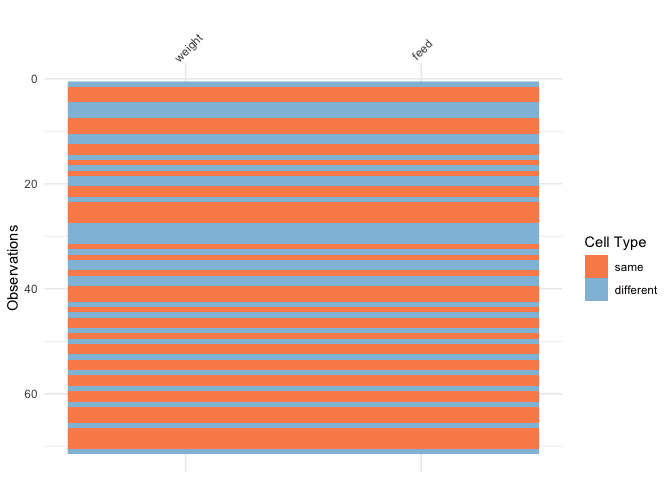Here the differences are marked in blue.

If you try and compare differences when the dimensions are different, you get an ugly error:

``````chickwts_diff_2 <- chickwts
chickwts_diff_2\$new_col <- chickwts_diff_2\$weight*2

vis_compare(chickwts, chickwts_diff_2)
# Error in vis_compare(chickwts, chickwts_diff_2) :
#   Dimensions of df1 and df2 are not the same. vis_compare requires dataframes of identical dimensions.``````

## Using `vis_expect()`

`vis_expect` visualises certain conditions or values in your data. For example, If you are not sure whether to expect values greater than 25 in your data (airquality), you could write: `vis_expect(airquality, ~.x>=25)`, and you can see if there are times where the values in your data are greater than or equal to 25:

``vis_expect(airquality, ~.x >= 25)``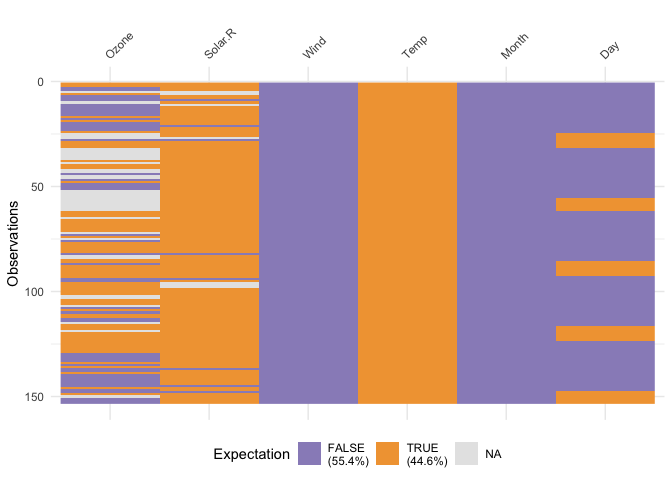This shows the proportion of times that there are values greater than 25, as well as the missings.

## Using `vis_cor()`

To make it easy to plot correlations of your data, use `vis_cor`:

``vis_cor(airquality)``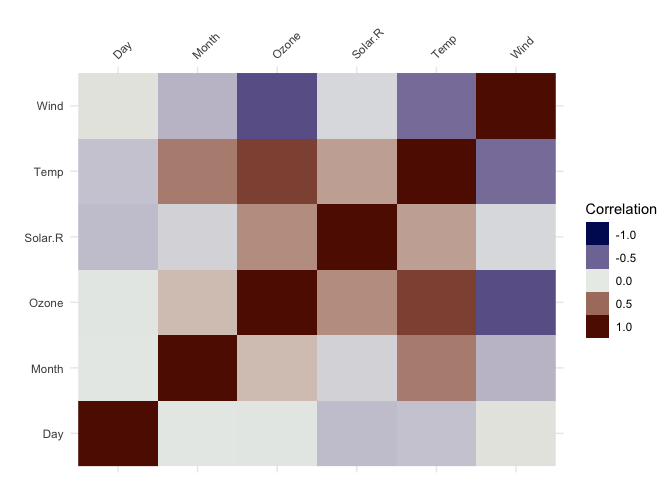## Using `vis_value`

`vis_value()` visualises the values of your data on a 0 to 1 scale.

``vis_value(airquality)``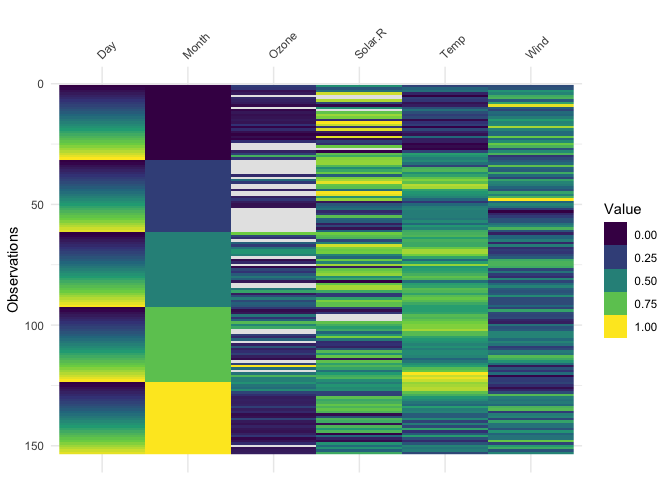It only works on numeric data, so you might get strange results if you are using factors:

``````library(ggplot2)
vis_value(iris)``````
``data input can only contain numeric values, please subset the data to the numeric values you would like. dplyr::select_if(data, is.numeric) can be helpful here!``

So you might need to subset the data beforehand like so:

``````library(dplyr)
#>
#> Attaching package: 'dplyr'
#> The following objects are masked from 'package:stats':
#>
#>     filter, lag
#> The following objects are masked from 'package:base':
#>
#>     intersect, setdiff, setequal, union

iris %>%
select_if(is.numeric) %>%
vis_value()``````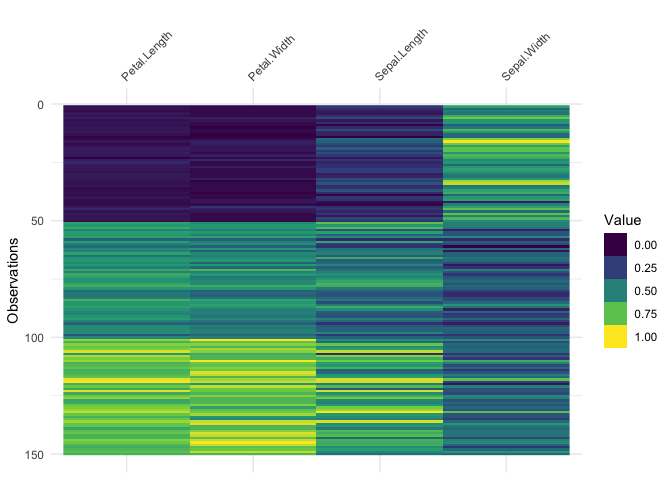## Using `vis_binary()`

`vis_binary()` visualises binary values. See below for use with example data, `dat_bin`

``vis_binary(dat_bin)``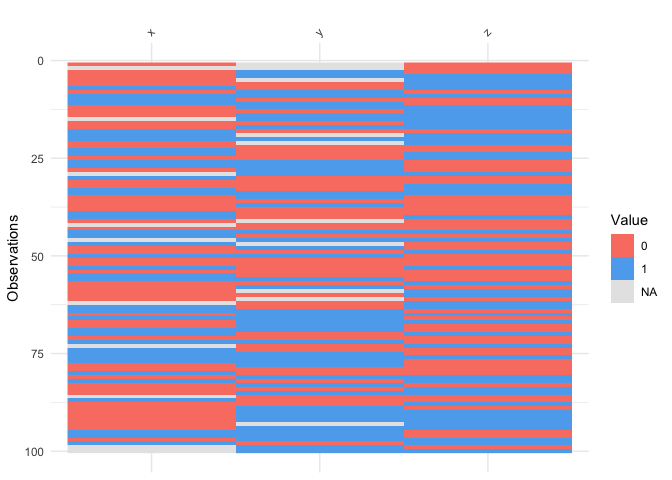If you don’t have only binary values a warning will be shown.

``vis_binary(airquality)``
``````Error in test_if_all_binary(data) :
data input can only contain binary values - this means either 0 or 1, or NA. Please subset the data to be binary values, or see ?vis_value.``````

## Using `vis_guess()`

`vis_guess()` takes a guess at what each cell is. It’s best illustrated using some messy data, which we’ll make here:

``````messy_vector <- c(TRUE,
T,
"TRUE",
"T",
"01/01/01",
"01/01/2001",
NA,
NaN,
"NA",
"Na",
"na",
"10",
10,
"10.1",
10.1,
"abc",
"\$%TG")

set.seed(2019-04-03-1106)
messy_df <- data.frame(var1 = messy_vector,
var2 = sample(messy_vector),
var3 = sample(messy_vector))``````
``````vis_guess(messy_df)
vis_dat(messy_df)``````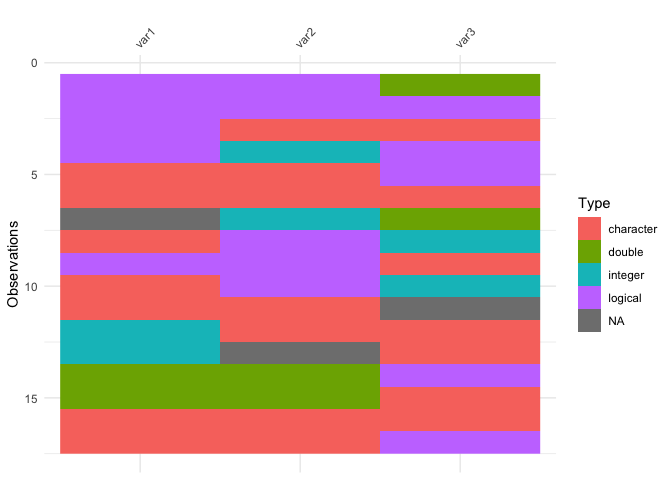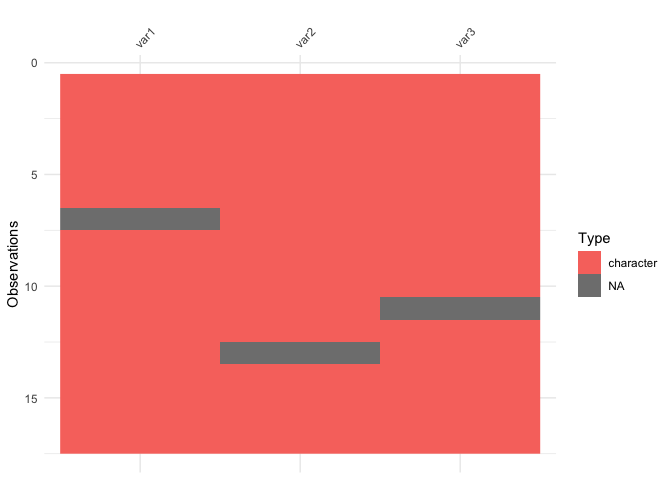So here we see that there are many different kinds of data in your dataframe. As an analyst this might be a depressing finding. We can see this comparison above.

# Thank yous

Thank you to Ivan Hanigan who first commented this suggestion after I made a blog post about an initial prototype `ggplot_missing`, and Jenny Bryan, whose tweet got me thinking about `vis_dat`, and for her code contributions that removed a lot of errors.

Thank you to Hadley Wickham for suggesting the use of the internals of `readr` to make `vis_guess` work. Thank you to Miles McBain for his suggestions on how to improve `vis_guess`. This resulted in making it at least 2-3 times faster. Thanks to Carson Sievert for writing the code that combined `plotly` with `visdat`, and for Noam Ross for suggesting this in the first place. Thank you also to Earo Wang and Stuart Lee for their help in getting capturing expressions in `vis_expect`.

Finally thank you to rOpenSci and it’s amazing onboarding process, this process has made visdat a much better package, thanks to the editor Noam Ross (@noamross), and the reviewers Sean Hughes (@seaaan) and Mara Averick (@batpigandme).